## Help stop the Arduino compiler outwitting me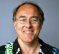Started by 2 months ago19 replieslatest reply 2 months ago136 views

It's me again (sorry). As a reminder, I'm a hardware design engineer, so my software knowledge is somewhat limited. I'm using the latest Arduino IDE 2 and I'm being outwitted by the Arduino compiler.

Here's the deal. I have one of the new Arduino Uno R4 boards (32-bit, 48MHz, lots of memory) and compared to the Uno R3 (8-bit, 16MHz clock, little memory).

I decided to compare the performance of integer and floating-point operations on both an R3 and an R4. Eventually I want to compare short ints (16 bits on both machines), regular ints (16-bit on the R3 and 32-bit on the R4), and long ints (32 bits on both platforms).

Of course I could look at the data sheet, but where's the fun in that?

I started with a program to test regular ints for the fundamental math operations (+, -, *, /, %).  A text version of the full program is here: http://www.clivemaxfield.com/area51/r3-vs-r4-test-...

The core of the program that runs the tests is as follows:

void RunTests ()
{
int tstVal;

for (int iTstTyp = 0; iTstTyp < NUM_TESTS; iTstTyp++)
{
TestTimes[iTstTyp].startTime = micros();

for (long iTsts = 0; iTsts < NUM_ITTERATIONS; iTsts++)
{
tstVal = 0x7692;
if      (iTstTyp == 0) {}
else if (iTstTyp == 1) {}
else if (iTstTyp == 2) {}
else if (iTstTyp == 3) tstVal += 0x0042;
else if (iTstTyp == 4) tstVal -= 0x0042;
else if (iTstTyp == 5) tstVal *= 0x0042;
else if (iTstTyp == 6) tstVal /= 0x0042;
else if (iTstTyp == 7) tstVal %= 0x0042;
}

TestTimes[iTstTyp].endTime = micros();
}
}

When I run this program on my R3, the results are as follows:

=====================
Test =  0  Start 2000012  End 2000016  Elapsed = 4
Test =  1  Start 2000020  End 2000024  Elapsed = 4
Test =  2  Start 2000028  End 2000032  Elapsed = 4
Test =  3  Start 2000036  End 2000036  Elapsed = 0
Test =  4  Start 2000040  End 2000044  Elapsed = 4
Test =  5  Start 2000048  End 2000052  Elapsed = 4
Test =  6  Start 2000056  End 2000060  Elapsed = 4
Test =  7  Start 2000064  End 2000068  Elapsed = 4

I'm running 10,000,000 iterations. Take Test 0 which is a simple compare to 0. Even if this takes only 1 clock cycle, I should still be looking at 10,000,000 / 16MHz = 650,000us -- NOT 4us.

Obviously the compiler is optimizing. It's a compiler from hell. For example, I modified the code to print out the resulting tstVal value for each test. In the case of the addition, I tried += 1 -- it gave me the result of 10,000,000 with the same elapsed time of 4us. Obviously it realized that it could pre-calculate the result.

I started to employ more complicated code -- the compiler realized if something isn't used, then there's no need to perform the operation. The trickier I tried to be, the more cunning the compiler.

Any thoughts (other than "you're an idiot") would be very much appreciated :-)

[ - ]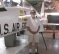A couple of things came to mind.

First: In the inner loop, with no testing at any test iteration, there are fewer "if" comparisons for the first test type to make than for the last test type. The first one compares equal and drops out the bottom of the structure. The last one has to make all of the comparisons before satisfying the condition. I would remove all of the "else" options and make every test type make the same number of comparisons.

Second: To remove the time for the "if" statements entirely, create a function for each test type. If you need to put those into a separate file to thwart some optimizations, do that. Make each function in the form: void TestTypeN() { ... }

So you have:

void TestType0(){}

void TestType1(){}

...

void TestType7(){}

Declare the a pointer to your functions as a type

typedef void(*testFunc)() testFunction;

And a table pointing to your functions:

testFunction test = {TestType0,TestType1, ... TestType7};

To use this mess:

for (int iTstTyp = 0; iTstTyp < NUM_TESTS; iTstTyp++)

{

TestTimes[iTstTyp].startTime = micros();

for (long iTsts = 0; iTsts < NUM_ITTERATIONS; iTsts++)

{

(*test[iTstType])();

}

TestTimes[iTstTyp].endTime = micros();

}

[ - ]Someone else said something similar -- this was one of my reasons for doing tests 0, 1, and 2 -- to determine the overhead of the if() and else-if() tests so I could subtract them from the final results -- but both of your ways are better.
[ - ]First things first, to force the compiler not to optimize loops away, define tstVal as volatile int. Does that produce reasonable results?

[ - ]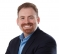My first thought as well. Just to fill in some details Max, when you declare a variable volatile, you're telling the compiler that the variable value could change at any time. Because the value could change at any time, the compiler won't apply optimizations to that variable. The compiler might see that your loop values never change, so it optimizes to the final result.

If you were to look at the disassembly for the loop, you would be able to review it and see what the compiler output is. (I don't think this is an option though within the Arduino IDE).

[ - ]Hi Jacob -- I've never even heard about volatile variables -- I learn something new every day!

[ - ]Thanks for the suggestion -- I will try this as soon as I get home tonight.

[ - ]Yup!  My guess also is to specify ‘volatile’

[ - ]How is it everyone knows about 'volatile' but me (LOL)?

[ - ]I know about 'Volatile' because I've been bitten by it enough times in the past ;-)

[ - ]But now it may be my new best friend (it's a funny old world :-)

[ - ]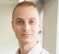As others have said, making testVal volatile might work, but another option could be moving each test to a separate source file. If each operator-assignment is separate from the main test loop, you could thwart the optimizer in seeing that no work is done by the function.

void test5(int* x) {*x *= 0x42;}

You could even make an array of pointers to these functions so calling them becomes am offset table and you have less impact from a delta.

[ - ]Do I look like the sort of man who can make an array of pointers? Now I'm going to have to go and re-read my book on "Understanding and Using C Pointers" https://www.amazon.com/gp/product/1449344186 (it made my head hurt the first time round)

[ - ]For a performance test Max your code currently has non-linear performance and this behaviour is within the timing start-end sequence.

To obtain results that can be used to compare different operations this isn't good. If you just wanted to compare the same operations between datatype runs (you said about trying differing int types) then this is okay.

I have tried to show a simple solution below using your existing if..else construct. A far nicer way is using function pointers in an array as suggested by wayden, but if your not used to function pointers can take a little to get your head round.

The solution below decides what test is to be run, then times around the for loop doing the iterations.

void RunTests ()
{
volatile int tstVal; /* volatile to avoid compiler optimisation */
/* variables are on the stack, define them first to be sure not done in the loop */
int iTstTyp;
long iTsts;

for (iTstTyp = 0; iTstTyp < NUM_TESTS; iTstTyp++)
{
/* switch-case or if-else sequences are non-linear in performance;
looping many times to remove timing noise won't account for this.
Best thing is to create either a linear construct such as an array
of function pointers indexed by the iTstTyp (as suggested by wayden
here), or to place the decision logic outside the loop as below. */
if ((iTstTyp == 0) || (iTstTyp == 1) || (iTstTyp == 2)) {
TestTimes[iTstTyp].startTime = micros();

for (iTsts = 0; iTsts < NUM_ITTERATIONS; iTsts++)
{
tstVal = 0x7692;
}
TestTimes[iTstTyp].endTime = micros();
} else if (iTstTyp == 3) {

TestTimes[iTstTyp].startTime = micros();

for (iTsts = 0; iTsts < NUM_ITTERATIONS; iTsts++)
{
tstVal = 0x7692;
tstVal += 0x0042;
}
TestTimes[iTstTyp].endTime = micros();

} /*... other test cases here */

}
}

[ - ]I see what you mean -- this was one of my reasons for doing tests 0, 1, and 2 -- to determine the overhead of the if() and else-if() tests so I could subtract them from the final results -- but your way is better.
[ - ]Another solution than separate compilation would be to print something depending on the calculation (Serial.println is separately compiled;

ex :

long cum = 0;

for (long iTsts = 0; iTsts < NUM_ITTERATIONS; iTsts++)
{...

// end of block

cum +=  tstVal + random(1); // random CANNOT be optimized out

}

Serial.println(cum);

This solution is less elegant than separate compilation, but is a little easier (one can forget one needs two separate files)

Edited : I am not sure volatile is enough (setting to a constant is invariant);

[ - ]I had started to move this way -- actually using the result as part of a Serial.print() -- because that way the compiler knows I want a result -- but it was still doing weird things -- the use of random() is interesting -- thanks for the suggestion.

[ - ]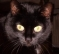Hello Max!

Definitely the compiler optimizes out the whole loop!

First please turn on all messages in f****ing Arduino: File --> Preferences --> Show verbose output during [x] compile [x] upload

Compiler warnings: All

The binary size of your sketch is 34304 bytes. If you comment out parts of your loop:

for (long iTsts = 0; iTsts < NUM_ITTERATIONS; iTsts++)

{

tstVal = 0x7692;

if      (iTstTyp == 0) {}

else if (iTstTyp == 1) {}

else if (iTstTyp == 2) {}

else if (iTstTyp == 3) tstVal += 0x0042;

//else if (iTstTyp == 4) tstVal -= 0x0042;

//else if (iTstTyp == 5) tstVal *= 0x0042;

//else if (iTstTyp == 6) tstVal /= 0x0042;

//else if (iTstTyp == 7) tstVal %= 0x0042;

}

it remains 34304 bytes! More to say?

The problem is likely one of the compiler flags:

arm-none-eabi-g++ -c -w -Os -g3 -fno-use-cxa-atexit -fno-rtti -fno-exceptions -nostdlib -DF_CPU=48000000 andmoreblablabla

-Os optimizes out your loop, -O0 would turn off optimization. Unfortunately these compiler flags cannot be changed easily in Arduino. This is defined in an text file called platform.txt

On my machine, for R4 this is hidden in Users\User\AppData\Local\Arduino15\packages\arduino\hardware\renesas_uno\1.0.2, but changing there does NOT change the compile process! I cannot find where in Arduino the compiler flags are set, very sad!

BUT! If you define

volatile int tstVal;

the code size of the binary increases to 34392 bytes and changes if you comment out single lines of the if-else part, indicating that the code is not optimized out.

This therefore should normally work as intended, unless there are more flaws.

Best regards,

Eric

[ - ]Maybe also interesting, if you move tstVal outside of the function RunTests() into global name space:

struct TestTime_t TestTimes[NUM_TESTS];

int tstVal;

then the codesize remains 34392 bytes, so the loop is NOT optimized away even WITHOUT volatile. The compiler then probably assumes that someone else may use tstVal.

But, if you now declare tstVal local to the module using the keyword static:

struct TestTime_t TestTimes[NUM_TESTS];

static int tstVal;

the code size is again 34304 bytes, so the loop is optimized away -- the compiler is quite a smart beast! To still prevent this you may use:

struct TestTime_t TestTimes[NUM_TESTS];

static volatile int tstVal;

This results even in a slightly bigger code size: 34400 bytes. This can only be explained by looking at the assembler output ... but for this you need to have control of the compiler flags. Local vars are on stack, global vars are not, so accessing differs. Have to look deeper into the processors architecture.

[ - ]